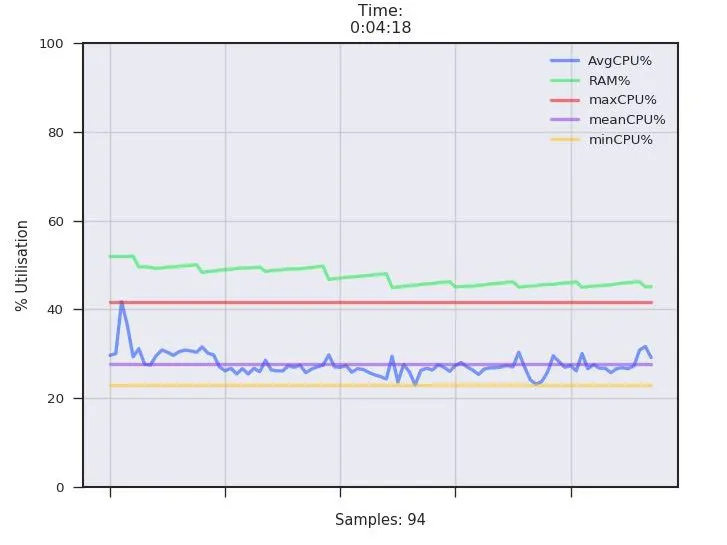# RPi3 Utilisation Monitor / Sensor Plotter

A CPU and Ram usage monitor, displays a live plot on port 8000, can be configured to plot anything the pi can measure.

IntermediateProtip997## Things used in this project

### Hardware componentsRaspberry Pi 3 Model B
×1

### Software apps and online services

 Python3

## Code

### PyMon

Python
A Python3 web app to monitor CPU and RAM %'s Vs time.
```import matplotlib
matplotlib.use('Agg')
from io import BytesIO
import base64
import matplotlib.pyplot as plt
import pandas as pd
import psutil
import seaborn as sns
from datetime import datetime
import time

application.config['SECRET_KEY'] = 'secret!'
socketio = SocketIO(application)

sns.set()
sns.set_context("paper")
sns.set_style("ticks", {'axes.facecolor': '#EAEAF2', 'axes.grid': True, 'grid.color': '.8', 'grid.linestyle': u'-'})
sns.set_palette("bright")
DataPointCount = 0
df = pd.DataFrame()
firstrequest = False
disconnectCount = 0
connecttime = time.time()

@socketio.on('connect', namespace='/')
def test_connect():
global disconnectCount, connecttime
disconnectCount = 0
emit('my response', {'data': 'Connected'})
connecttime = time.time()
print('{} connected    @ {}'.format(ip, datetime.now().strftime("%H:%M:%S on %d/%m/%y")))

@socketio.on('disconnect', namespace='/')
def test_disconnect():
global disconnectCount, DataPointCount, firstrequest, df
disconnectCount += 1
print('{} disconnected @ {}'.format(ip, datetime.now().strftime("%H:%M:%S on %d/%m/%y")))

@application.route('/')
def index():
global width, DataPointCount, df, clock, startClock, firstrequest, connecttime
width = 2
if not firstrequest:
firstrequest = True
startClock = datetime.now()
clock = datetime.now()

while True:
try:
# If clock var is not equal to actual clock, run this...
if clock != datetime.now():
TD = datetime.now() - startClock
CpuVal = psutil.cpu_percent(interval=None, percpu=False)
RamVal = psutil.virtual_memory()
df2 = pd.DataFrame({'AvgCPU%': CpuVal, 'RAM%': RamVal,
'Time':datetime.now().strftime("%S")},
index=[DataPointCount])
df = df.append(df2)
df['meanCPU%'] = df['AvgCPU%'].mean()
df['minCPU%'] = df['AvgCPU%'].min()
df['maxCPU%'] = df['AvgCPU%'].max()
df.set_index("Time", drop=True, inplace=True)

if DataPointCount > 2:
plt.figure()
if DataPointCount % 500 == 0:
width = width / 1.3
if width < 0.5:
width = 0.5

df.plot(ylim=(0, 100), linewidth=width, alpha=0.5)
plt.Axes.set_autoscalex_on(plt, True)
plt.minorticks_off()
TD = str(TD)
plt.xlabel('Samples: {}'.format(DataPointCount))
plt.ylabel("% Utilisation")
TD = TD.split('.')
plt.title("Time:\n{}".format(TD))
img_base64 = BytesIO()
plt.savefig(img_base64, format='png', dpi=120)
img = base64.b64encode(img_base64.getvalue())
img = str(img)
img = img[2:-1]
DataPointCount += 1
if time.time() - connecttime > 10:
DataPointCount = 0
firstrequest = False
df = pd.DataFrame()
connecttime = time.time()
plt.close('all')

return render_template('index.html', value=img)

DataPointCount += 1
plt.close('all')

except(KeyboardInterrupt, SystemExit):
return 0

if __name__ == '__main__':
print('Server Up !')
socketio.run(application, host='0.0.0.0', port=8000)
```

### index.html

HTML
The index file for the web app (Required, Place in a folder called templates, beside app)
```<!DOCTYPE html>
<html lang="en">
<script src="https://code.jquery.com/jquery-3.3.1.slim.min.js" integrity="sha384-q8i/X+965DzO0rT7abK41JStQIAqVgRVzpbzo5smXKp4YfRvH+8abtTE1Pi6jizo" crossorigin="anonymous"></script>
<script type="text/javascript" src="//cdnjs.cloudflare.com/ajax/libs/socket.io/1.3.6/socket.io.min.js"></script>
<script type="text/javascript" charset="utf-8">
var socket = io.connect('http://' + document.domain + ':' + location.port + '/');
socket.on('my response', function(msg) {
\$('#log').append('<p>Received: ' + msg.data + '</p>');
});
\$('form#emit').submit(function(event) {
socket.emit('my event', {data: \$('#emit_data').val()});
return false;
});
});
</script>

<meta charset="utf-8">
<meta name="viewport" content="width=device-width, initial-scale=1, shrink-to-fit=yes">
<meta name="description" content="">
<meta name="author" content="">
<meta http-equiv="refresh" content="1">
<!-- ^^^ auto refresh ^^^ -->
<!-- Latest compiled and minified CSS -->
href="https://maxcdn.bootstrapcdn.com/bootstrap/4.1.0/css/bootstrap.min.css">
<title>Python Monitor</title>
<body>
<div class="row">
<div class="mx-auto text-center">
<img src="data:image/png;base64,{{ value }}" alt="PyMon">
</div>
</div>
</body>
</html>
```

## Credits

### Bobby Leonard

6 projects • 21 followers
Making and breaking computers and electronics. Coding, Networking, Data Sec etc etc. Physics fan also.